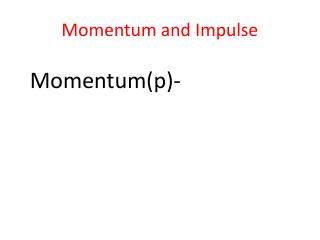DownloadDownload PresentationMomentum and Impulse

# Momentum and Impulse

Download Presentation## Momentum and Impulse

- - - - - - - - - - - - - - - - - - - - - - - - - - - E N D - - - - - - - - - - - - - - - - - - - - - - - - - - -
##### Presentation Transcript

1. Momentum and Impulse Momentum(p)-

2. Momentum and Impulse Momentum- Newton referred to it as the quantity of motion

3. Momentum and Impulse Momentum- It is defined mathematically as the product of mass and velocity of an object

4. Momentum and Impulse Momentum-

5. Momentum and Impulse Momentum is a vector quantity. The momentum vector points in the same direction as the velocity vector.

6. Momentum and Impulse Example – What is the momentum of a puck with a mass of 2.0kg sliding to the right on the x axis at 2.0m/s

7. Momentum and Impulse Example –

8. Momentum and Impulse The momentum of a system of objects is equal to the vector sum of the momenta of the individual objects.

9. Momentum and Impulse Example – What is the momentum of a system consisting of puck with a mass of 2.0kg sliding to the right along the x axis at 2m/s, and another puck with a mass of 1.0kg sliding to the left along the x axis at 5.0m/s

10. Momentum and Impulse Example –

11. Momentum and Impulse Impulse (J) is defined as the change in momentum

12. Momentum and Impulse Impulse (J) is also equal to the average force acting on an object multiplied by the time interval during which it is acting.

13. Momentum and Impulse Example – a ball with a mass of 0.50kg and moving with a velocity of 10.0m/s strikes a wall, and bounces off in the opposite direction at 5.0 m/s. Consider the initial velocity to be positive. Calculate the impulse given to the ball If the contact with the wall lasted for 0.01s what was the magnitude of the average force exerted on the ball?

14. Momentum and Impulse Example a.

15. Momentum and Impulse Example a. The negative sign indicates that the force acted in the direction opposing the original motion

16. Momentum and Impulse Summary: